自监督对比损失和监督对比损失的对比

Supervised Contrastive Learning这篇论文在有监督学习、交叉熵损失与有监督对比损失之间进行了大量的讨论，以更好地实现图像表示和分类任务。让我们深入了解一下这篇论文的内容。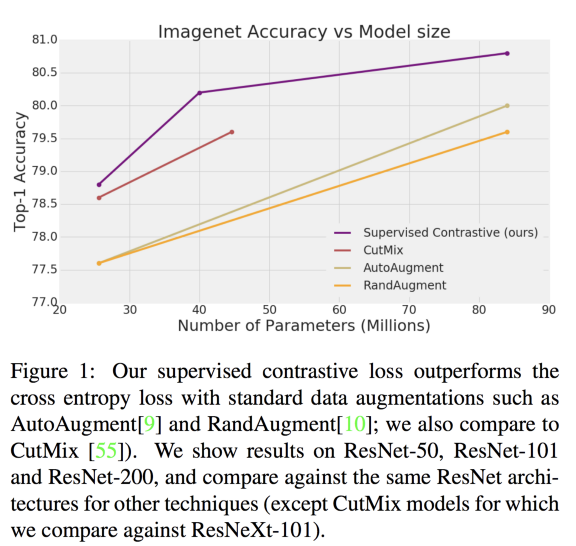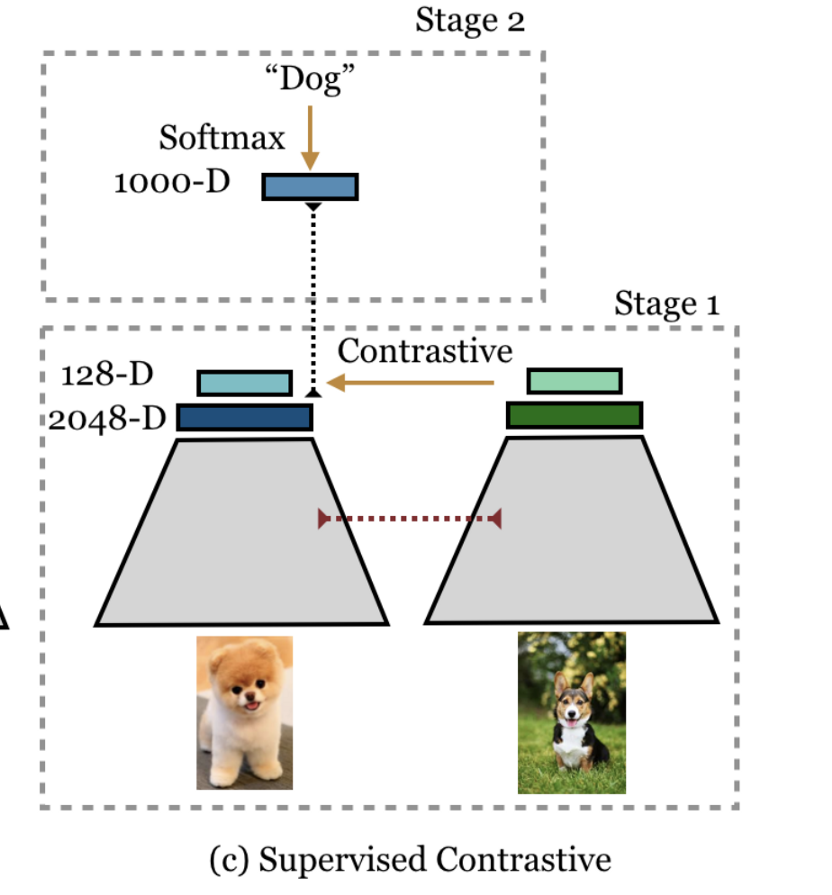Code

self.encoder = resnet50()

def forward(self, x):
feat = self.encoder(x)
#需要对128向量进行标准化
return feat

• 使用对比损失的训练集（两种变化）

• 冻结参数，然后使用softmax损失在线性层上学习分类器。（来自论文的做法）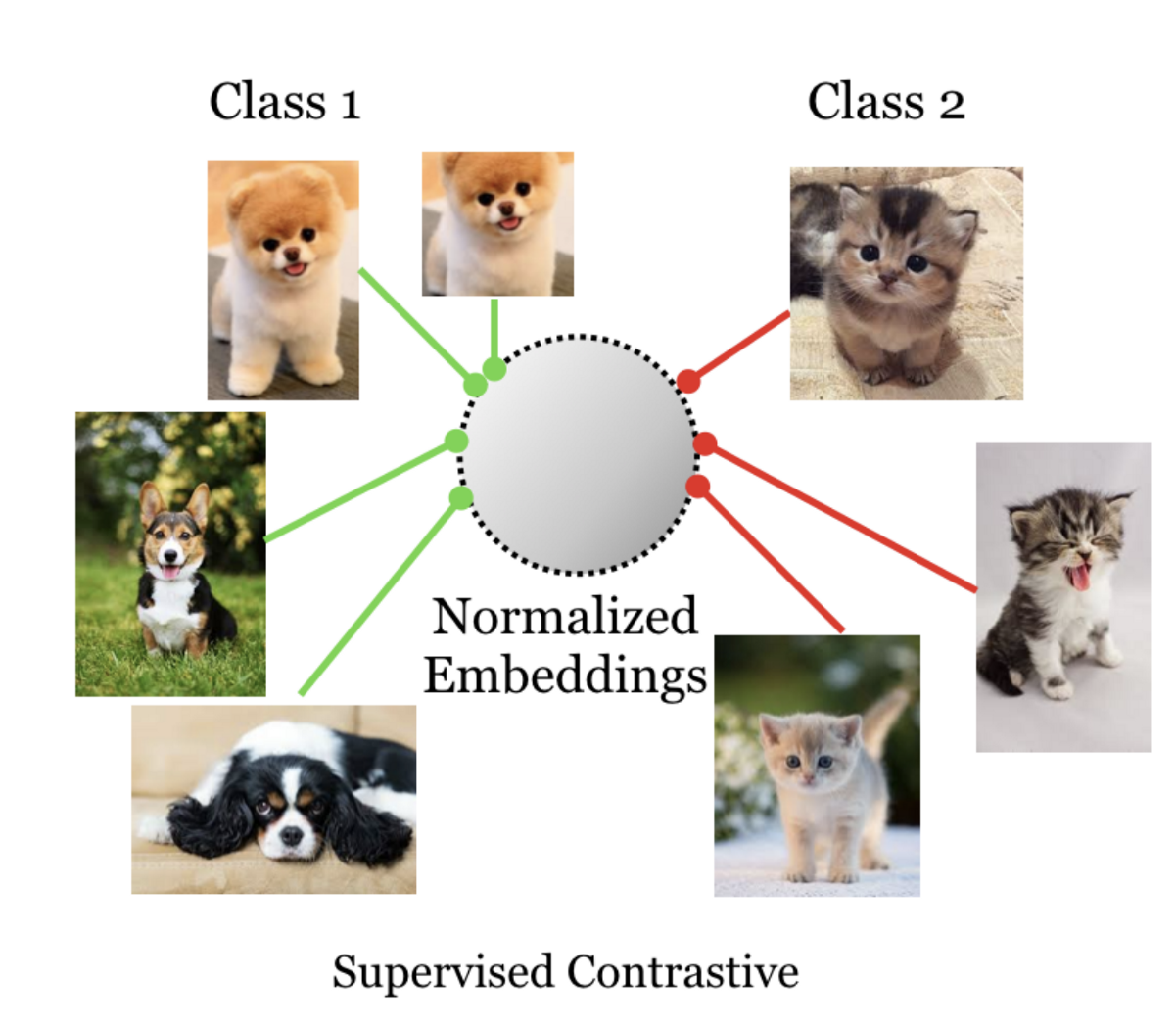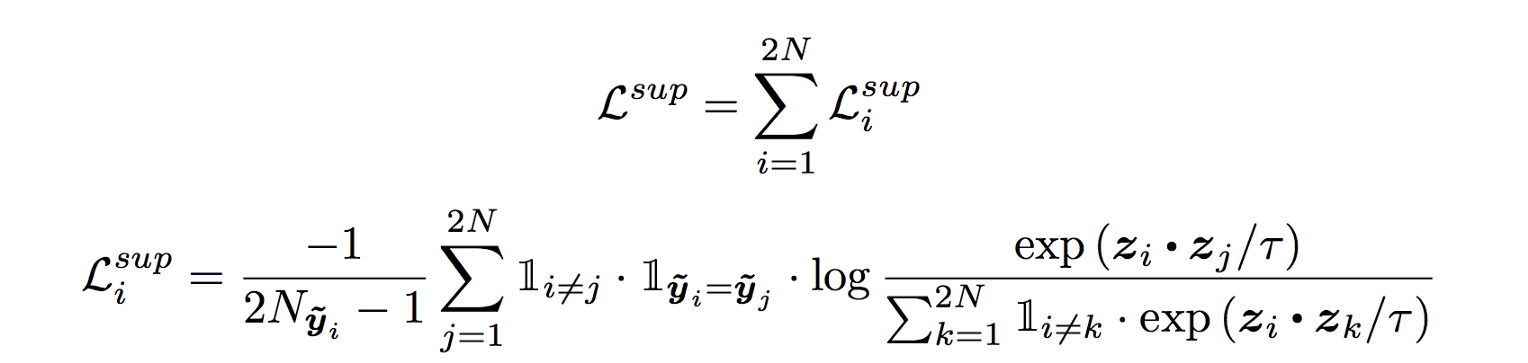#尝试理解下面的代码

import numpy as np
v = np.random.randn(128)
v = v/np.linalg.norm(v)
print(np.dot(v,v))
print(np.linalg.norm(v))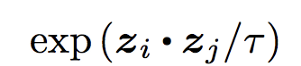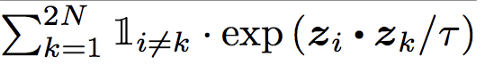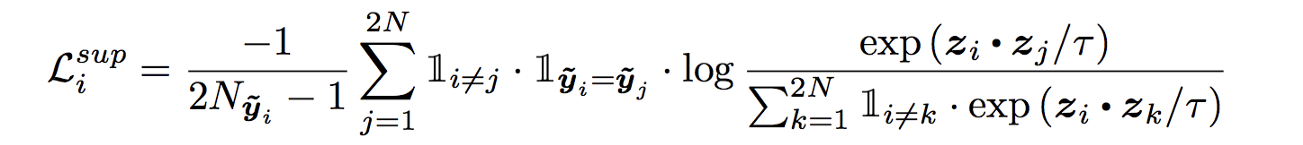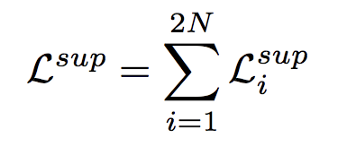8=4x2的原因是我们对每个图像总是有一个对比度，因此需要相应地编写一个数据加载程序。

#batch大小
bs = 4temperature = 0.07

anchor_feature = contrast_feature

anchor_dot_contrast = torch.div(
torch.matmul(anchor_feature, contrast_feature.T),
temperature)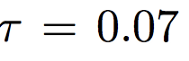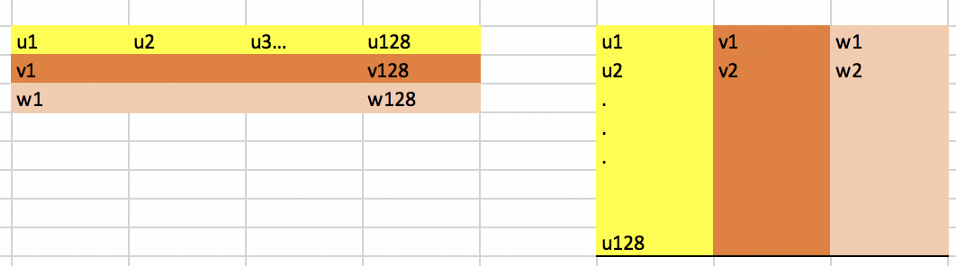anchor_feature=3x128和contrast_feature=128x3，结果为3x3，如下所示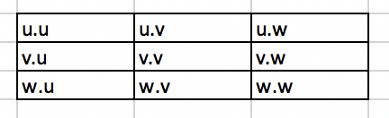#bs 1 和 dim 2 意味着 2*1x2
features = torch.randn(2, 2)

temperature = 0.07
contrast_feature  = features
anchor_feature = contrast_feature
anchor_dot_contrast = torch.div(
torch.matmul(anchor_feature, contrast_feature.T),
temperature)
print('anchor_dot_contrast=\n{}'.format(anchor_dot_contrast))

logits_max, _ = torch.max(anchor_dot_contrast, dim=1, keepdim=True)
print('logits_max = {}'.format(logits_max))
logits = anchor_dot_contrast - logits_max.detach()
print(' logits = {}'.format(logits))

#输出看看对角线发生了什么

anchor_dot_contrast=
tensor([[128.8697, -12.0467],
[-12.0467,  50.5816]])
logits_max = tensor([[128.8697],
[ 50.5816]])
logits = tensor([[   0.0000, -140.9164],
[ -62.6283,    0.0000]])

bs = 4
print('batch size', bs)
temperature = 0.07
labels = torch.randint(4, (1,4))
print('labels', labels)

#对它进行硬编码，以使其更容易理解
contrast_count = 2
anchor_count = contrast_count

#屏蔽self-contrast的情况
1,
torch.arange(bs * anchor_count).view(-1, 1),
0
)

batch size 4
labels tensor([[3, 0, 2, 3]])

#以上的意思是在这批4个品种的葡萄中，我们有3,0,2,3个标签。以防你们忘了我们在这里只做了一次对比所以我们会有3_c 0_c 2_c 3_c作为输入批处理中的对比。

tensor([[0., 1., 1., 1., 1., 1., 1., 1.],
[1., 0., 1., 1., 1., 1., 1., 1.],
[1., 1., 0., 1., 1., 1., 1., 1.],
[1., 1., 1., 0., 1., 1., 1., 1.],
[1., 1., 1., 1., 0., 1., 1., 1.],
[1., 1., 1., 1., 1., 0., 1., 1.],
[1., 1., 1., 1., 1., 1., 0., 1.],
[1., 1., 1., 1., 1., 1., 1., 0.]])

# 所以我们的标签就是标签张量([[3,0,2,3]])
# 我重新命名它们是为了更好地理解张量([[3_1,0_1,2_1,3_2]])

tensor([[0., 0., 0., 1., 1., 0., 0., 1.],
[0., 0., 0., 0., 0., 1., 0., 0.],
[0., 0., 0., 0., 0., 0., 1., 0.],
[1., 0., 0., 0., 1., 0., 0., 1.],
[1., 0., 0., 1., 0., 0., 0., 1.],
[0., 1., 0., 0., 0., 0., 0., 0.],
[0., 0., 1., 0., 0., 0., 0., 0.],
[1., 0., 0., 1., 1., 0., 0., 0.]])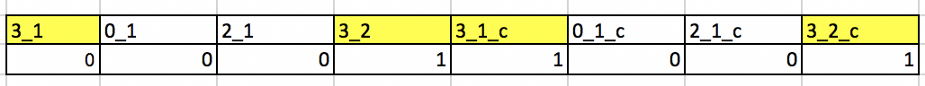logits = anchor_dot_contrast — logits_max.detach()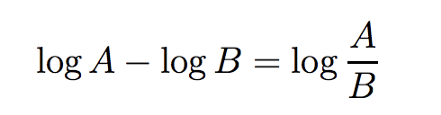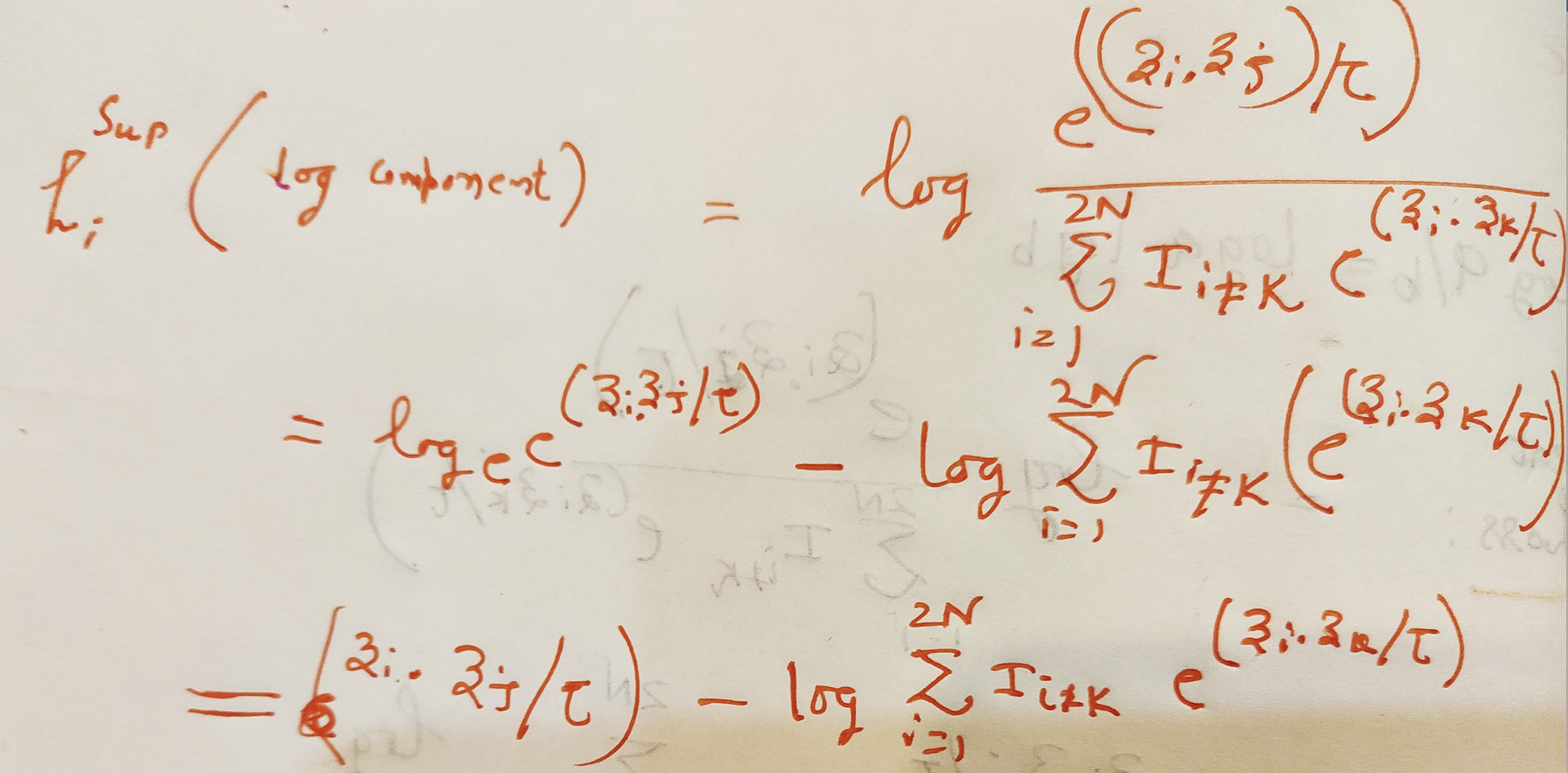#上述等式的第二部分等于torch.log(exp_logits.sum(1, keepdim=True))

log_prob = logits - torch.log(exp_logits.sum(1, keepdim=True))

# 计算对数似然的均值

# 损失
loss = - mean_log_prob_pos

loss = loss.view(anchor_count, 4).mean()
print('19. loss {}'.format(loss))

•  : Supervised Contrastive Learning
•  : Florian Schroff, Dmitry Kalenichenko, and James Philbin. Facenet: A unified embedding for face recognition and clustering. In Proceedings of the IEEE conference on computer vision and pattern recognition, pages 815–823, 2015.
•  : A Simple Framework for Contrastive Learning of Visual Representations, Ting Chen, Simon Kornblith Mohammad Norouzi, Geoffrey Hinton